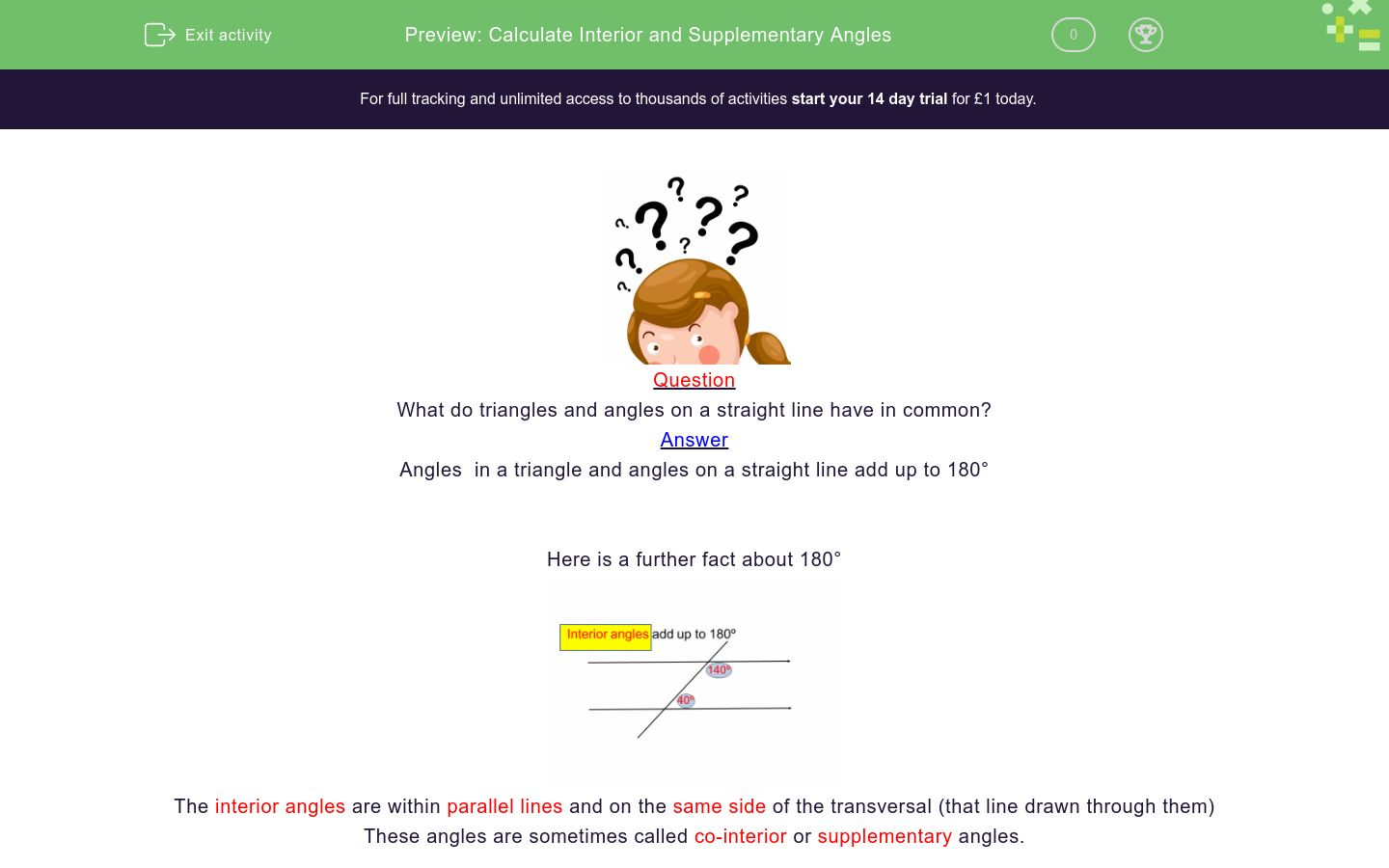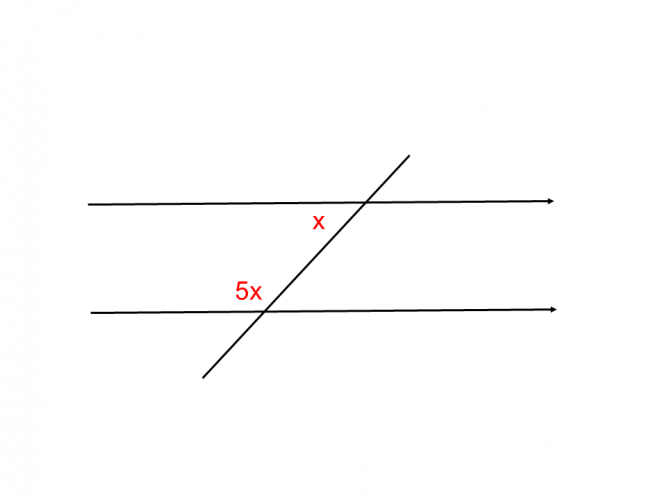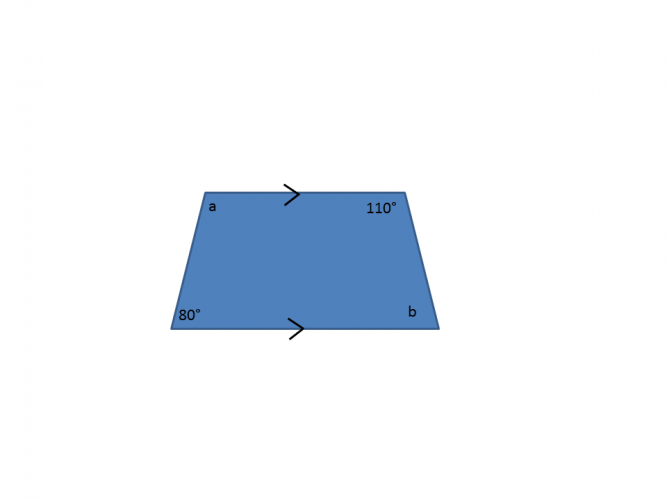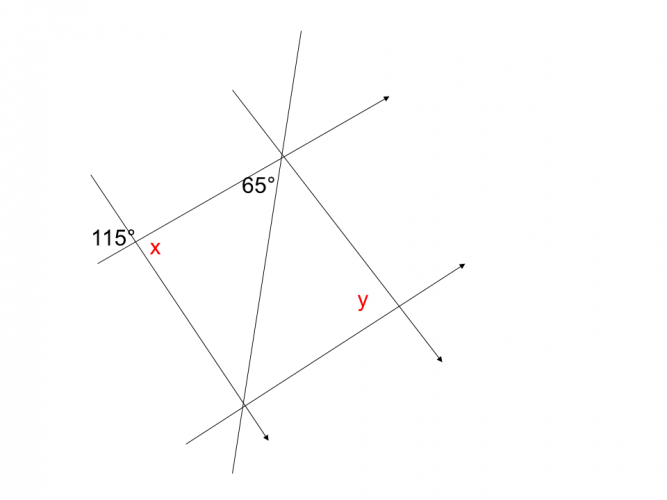# Calculate Interior and Supplementary Angles

In this worksheet, students will calculate interior angles within parallel lines.Key stage:  KS 4

GCSE Subjects:   Maths

GCSE Boards:   AQA, Eduqas, Pearson Edexcel, OCR

Curriculum topic:   Geometry and Measures, Basic Geometry

Curriculum subtopic:   Properties and Constructions, Angles

Difficulty level:### QUESTION 1 of 10Question

What do triangles and angles on a straight line have in common?

Angles  in a triangle and angles on a straight line add up to 180°

Here is a further fact about 180°The interior angles are within parallel lines and on the same side of the transversal (that line drawn through them)

These angles are sometimes called co-interior or supplementary angles.

There are very few numbers we have to remember in maths 180 is one of them.

Great stuff three facts for the price of one.

To calculate a missing interior angle just subtract the angle given from 180°Which sets of angles are interior angles?

h and d

g and h

g and c

a and bFind the value of x.61° 71° 81° The value of x is61° 71° 81° The value of x is216° 180° 144° 134° interior angles add up to the value of y isWhat is the value of x?What is the value of x?

45°

55°

40°

30°45°

55°

40°

30°45°

55°

40°

30°115° 65° 80° Angle x = Angle y =
• Question 1Which sets of angles are interior angles?

h and d
g and c
EDDIE SAYS
Interior is the clue here. Look inside the parallel lines. The two angles are on the same side of the transversal. h and d would add up to 180° so would g and c
• Question 2Find the value of x.

52
EDDIE SAYS
The magic number is 180, so a little bit of work to do here. 180 - 128 = 52° Always show your working out as this is where the marks come from.
• Question 361° 71° 81° The value of x is
EDDIE SAYS
A simple subtraction is all that is required. 180 - 109 = 71° Have you noticed that interior angles make a C shape or a backward C shape within the parallel lines? You could use this to remind you that a Calculation is required.
• Question 4EDDIE SAYS
180 - 132 = 48° Top tip. have you noticed that one angle is obtuse and the other acute. If you get two acute or two obtuse then you have made a mistake somewhere.
• Question 5216° 180° 144° 134° interior angles add up to the value of y is
EDDIE SAYS
There is no other explanation really just a simple subtraction. All you have to remember is that the interior angles add up to 180° 180 - 36 = 144°
• Question 6What is the value of x?

74
EDDIE SAYS
Are you getting the hang of this now? 180 - 106 = 74° What else could there be to it?
• Question 7What is the value of x?

30°
EDDIE SAYS
Oh no...whats this. I hope you are not panicking. We have all the knowledge that we need. We know that the angles add up to 180° We know that there are 5x + x = 6x altogether. Let's just share 180 between 6 lots of x. 180 ÷ 6 = 30°
• Question 8EDDIE SAYS
No problems this time. Bring it on. There is 8 lots of y and we are going to share it between 180° 180 ÷ 8 = 22.5°
• Question 9EDDIE SAYS
A trapezium has one set of parallel sides. Often sneaky teachers will put an interior angles question into one of these. No worries to us though, we can see that there are two sets of interior angles. To find angle a. 180 - 80 = 100° To find angle b, 180 - 110 = 70° A teacher will have to be a little more sneaky than that.
• Question 10115° 65° 80° Angle x = Angle y =
EDDIE SAYS
Nice try teacher, but no worries for us. One extra line isn\'t going to confuse us is it. You can see here that there are two sets of interior angles but only one calculation required. x is interior with 65° as is y. 180 - 65 = 115°
---- OR ----

Sign up for a £1 trial so you can track and measure your child's progress on this activity.

### What is EdPlace?

We're your National Curriculum aligned online education content provider helping each child succeed in English, maths and science from year 1 to GCSE. With an EdPlace account you’ll be able to track and measure progress, helping each child achieve their best. We build confidence and attainment by personalising each child’s learning at a level that suits them.

Get started